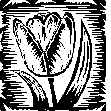Plant Physiology (Biology 327)  - Dr. Stephen G. Saupe;  College of St. Benedict/ St. John's University;  Biology Department; Collegeville, MN  56321; (320) 363 - 2782; (320) 363 - 3202, fax;    ssaupe@csbsju.edu

Preparing a Hofler Diagram

(note:  this lab is 'experimental.'  It may need some fine tuning)

In this exercise we will use our Gravimetric data to prepare a Hofler diagram to represent the changes in water potential, osmotic potential and pressure potential that occur in the potato tuber as they absorb water.

Protocol:

1.  Record the temperature of the samples in table 1.  Convert to K.

2.  Complete Table 2.

• At equilibrium, the water potential of the core will equal the water potential of the sucrose solution.  Calculate with the van't Hoff equation (see Chardakov lab)

• Transfer the equilibrium weights (=final weight) and initial weights of the cores from the Gravimetric experiment for columns 3 & 4, respectively.

• The relative cell volume (column 5) will equal 1.0 for a solution that has the same Y as the tuber.  In other words, when the solution and the tuber have the same water potential there will be no net change in size of the tuber cells since there will be no net uptake/loss of water.  However, cells gaining weight will have a larger relative cell volume while those loosing weight will have a smaller relative cell volume.

• Calculate Ψs (column 6) at equilibrium by dividing the solute potential (Ψs; measured with FP apparatus) by the relative cell volume.  This will correct for changes in Ψs due to water loss/gain.

• Calculate Ψp (colulmn 7) from the expression:  Ψw = Ψs + Ψp

2.      Plot a graph of relative cell volume vs. [sucrose]

3.      Prepare on a single graph the following plots: water potential vs. relative cell volume; osmotic potential vs. relative cell volume; and pressure potential vs. relative cell volume.

 Table 1.  Incubation Temperature temperature ( C) temperature (K)

 Table 2:  Potato Tuber Data [Sucrose] (molality) Ψ of the core at equilibrium Equilibrium (final) Weight (g) Initial Weight (g) Relative cell volume (equilibrium weight / initial weight) Equilibrium Ψs (corrected for cell volume) Pressure Potential (Ψp ) 0 0.1 0.2 0.3 0.4 0.5 0.6 0.7

Data/Analysis:

1. How does the graph we prepared compare to the expected plot?

References:

 | Top | SGS Home | CSB/SJU Home | Biology Dept | Biol 327 Home | Disclaimer |

Last updated:  01/07/2009     � Copyright  by SG Saupe